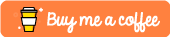#Data Structures - Hash Tables For Beginners

This tutorial will use Python 3.6 to convey the concepts covered.

So, in this tutorial, we are going to be looking at Hash Tables and how they can be used within our applications for fame and fortune. By the end of this tutorial, you should have a solid grasp of these Hash Tables and how they work under the covers.

# An Introduction

So, the first place we should start with is, what are hash tables? Hash Tables in Computer Science are a data structure that allow you to store key value pairs in such a way that allows for very quick look-up.

By knowing how hashmaps work fundamentally, we can drastically improve the performance of certain parts of our applications by leveraging this knowledge.

## An Example

Let’s take for example a hypothetical example in which we filter a stream of incoming tweets. Every time we receive a tweet, we need to check if we care about the author of that particular tweet.

Now, we could use an array to store a list of authors we care about and then enumerate through this list every time we get a new tweet in.

``````tweet_author = "realpython"
authors = ["elliot_f", "golangweekly", "realpython"]

for author in authors:
if author == tweet_author:

This would certainly work. However, what happens when we increase the number of authors we want to keep track of?

For each new author, we’ll essentially be doing 1 more comparison per tweet. If we are tracking 100 authors, and we get a tweet from someone we don’t follow, then we have done 100 unnecessary comparisons. Scale this up for the hundreds of millions of tweets that go out every day and we’ve done a tonne of unnecessary work.

### Using Hashmaps

We can improve the performance of our code above through the use of a hashmap.

Every time a new tweet comes in, we can take the tweet_author string and compute a hash. We can then almost instantly check to see if this hash matches something within our hashmap in `O(1)` time complexity and then decide what to do with that tweet if it is from one of the authors we are following:

``````tweet_author = "golangweekly"
authors = {"elliot_f":True, "realpython":True, "golangweekly":True}

if authors[tweet_author]:

With this implementation, if we receive a tweet from an author that we don’t follow, we are only performing one simple hashing computation and a lookup to check. This will drastically improve the speed at which our code can execute at scale.

# Performance

Good Hash Tables with minimal collisions allow you to perform inserts, finds and deletes in a constant amount of time, this can be expressed as `O(1)` in big-O notation.

This constant timing makes them incredibly valuable data-structures when compared to the likes of Arrays for instance. If we were tasked with the job of finding an element in `array`, we would typically have to iterate through all elements of that `array` before we found what we were looking for. Depending on the size of our `array`, this could be a fairly time-consuming task.

## Fill Factor

When we talk about hash tables and their implementation, we have to consider the `Fill Factor` of that particular hash table.

Hash tables with a high `Fill Factor` will tend to have a high number of collisions and as the `Fill Factor` of your hash table increases, the performance of said hash table starts to degrade.

# Hashing Algorithms

When designing a hash table, we need to come up with a good hashing algorithm that meets the following basic requirements:

• It must be efficient.
• It must compute the same hash value for equal objects.
• It should uniformly distribute our Hashes so that we don’t have clusters of hashes.
• It should have a low hash collision factor.

Typically, in languages like Python, these Hashing algorithms are provided for you and differ depending on the type of data you are hashing. However, they tend to give you the ability to come up with your own hashing algorithms should you wish.

# A Simple Example in Python

Now that we have covered some of the basic theory underlying Hash Tables, let’s have a look at how we would use Hash Tables within our Python applications.

We’ll be using a Python `dict` data type in this example as the `dict` is an implementation of a hash table.

``````# we can initialize an empty dict using curly braces like so
hash_table = {}
# next, we append an key which will be hashed, and a value
hash_table["random"] = "string"
# we can then retrieve the value of our hashed string like so
# the hashing of "random" takes O(1) time
print(hash_table["random"])``````

# Conclusion

I hope you found this tutorial useful! If you require further assistance then please let me know in the comments section below or by tweeting me @Elliot_F.